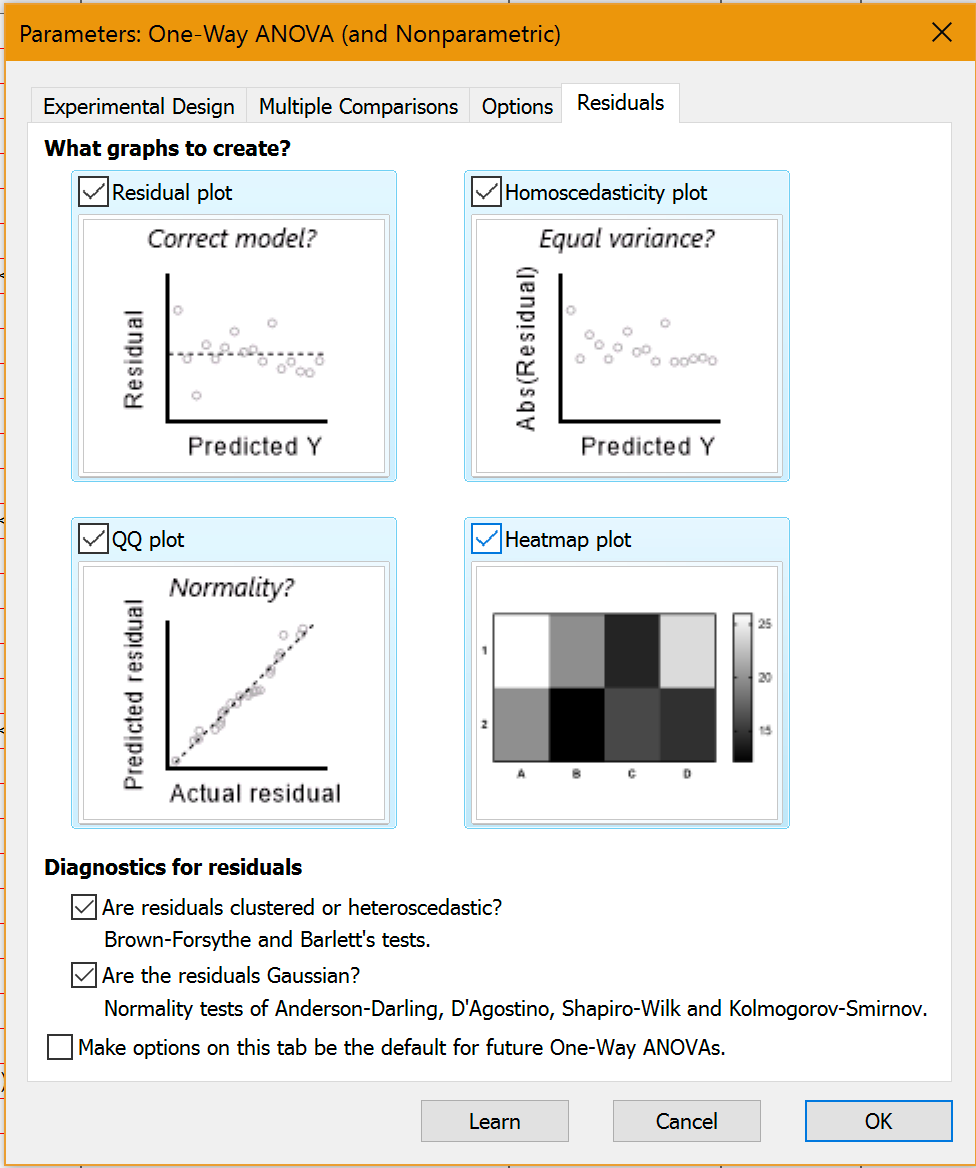## Please enable JavaScript to view this site.

 More analysis choices: Regression

Plot residuals and test them for normality

A residual is the difference between the actual and predicted value of Y. Prism 7 let you plot one kind of residuals from regression.

Prism 8 expands this to tabulate residuals also from ANOVA (one-, two- or three-way), t tests and multiple regression. For each of these analyses, test for normality of residuals in four ways and plot the residuals in four ways (including a QQ plot).Poisson regression

When the Y values represent an actual number of counts of objects or events (not normalized in any way), the residuals are not expected to follow a  Gaussian distribution, but  rather to follow a Poisson distribution.

Prism now lets you specify that you expect Poisson residuals for both nonlinear and multiple regression, and the calculations are done accordingly.

Logistic regression

When the outcome variable (Y values) are binary (yes/no, 1/0, alive/dead, etc.), you can use logistic regression to fit a model to your data. New in Prism 8.3.0, you can now perform simple logistic regression with a single predictor variable (X variable), or multiple logistic regression with many predictor variables.

More built-in equations for nonlinear regression

Pade(1,1) approximant equation, useful for interpolating when the standard curve plateaus at large concentrations (it is similar to a rectangular hyperbola).

Family of growth equations. These are used for everything from the growth of cell number in culture to the growth of economies. Equations offered are  exponential growth, exponential plateau, Gompertz, logistic, and beta (growth then decay).

Several forms of the linear quadratic equation used to model cell death after exposure to radiation.

Hinge function. It is the same as segmental linear regression, except that the two lines connect with a gentle curve instead of a hard angle.

Fit straight lines to two data sets and find the intersection point and both slopes.

In Prism 8.2, we expanded our equation library such that all dose-response equations now have forms for when X represents concentration and when X represents log(concentration).

Asymmetrical (profile likelihood) confidence intervals

Computing asymmetrical profile likelihood confidence intervals is 2-3 times faster.

With difficult equations, Prism sometimes reported "???" rather than a value for one or both of the confidence limits. This can still happen, but much less often.

New choices

When you choose to remove outliers in nonlinear regression, you can now ask Prism to create a results table with the "clean" data (without outliers).

Detect "Unstable" parameters in nonlinear regression: a new "Prism Labs" feature introduced in Prism 8.2 as an alternative to the method Prism uses to detect bad fits. Earlier versions detected "ambiguous fits". With the optional setting (on the Confidence tab of the nonlinear regression), Prism detects "unstable" parameters. In our experience, this method is better as the "ambiguous' method sometimes doesn't show results in cases where the new method can.